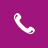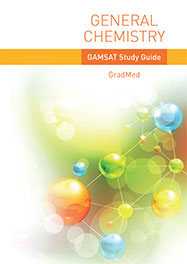020 7584 5818# General Chemistry

## Syllabus

• Basics of nuclear structure and hybridisation
• Bonding and structure, H-bonding in organic systems and electronegativity
• Oxidation number and usage in inorganic and organic chemistry
• Equilibria, Le Chatelier’s principle and its applications
• Solubility and Ksp calculations
• Solubility curves and phase diagrams
• Depression of freezing point and general colligative properties
• Acid Base equilibria, application to proteins and other biological systems, buffering, pKa usage and Indicators
• Buffering in the bloodstream
• Rates – the rate equation and determination of rate law
• Relation of rate law to mechanism
• Arrhenius equation, activation energy, relationship to biological mechanism
• Enzyme kinetics and development and use of Michaelis Menten equation
• Raoult’s law
• Entropy as a concept and Gibbs Free Energy
• Use of ΔG=ΔH-TΔS to predict reaction
• Chemical potential and application to biological systems
• Gibbs Free Energy profiles
• Osmotic pressure, Osmolarity and finding protein RMM
• Redox equilibria introduction
• Use of electrode potentials in predicting reaction, Nearnst equation, Electrode potentials in biological systems
• Stiff Diagrams

A range of other degree level areas are introduced, and application to simulated questions will enforce concepts, as they are covered on the course